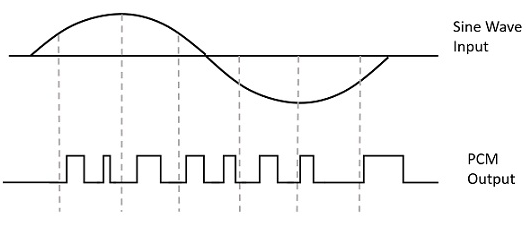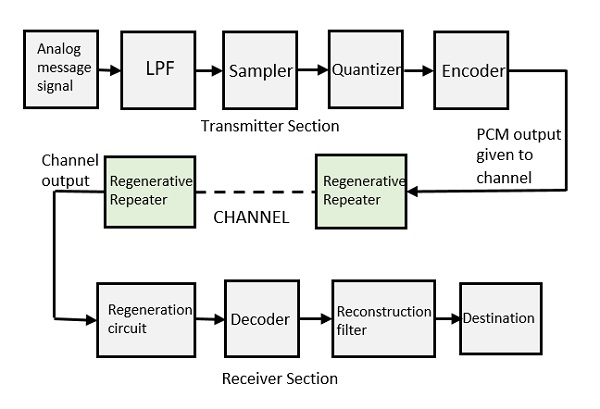# Digital Modulation

So far we have gone through different modulation techniques. The one remaining is digital modulation, which falls under the classification of pulse modulation. Digital modulation has Pulse Code Modulation (PCM) as the main classification. It further gets processed to delta modulation and ADM.

## Pulse Code Modulation

A signal is Pulse Code modulated to convert its analog information into a binary sequence, i.e., 1s and 0s. The output of a Pulse Code Modulation (PCM) will resemble a binary sequence. The following figure shows an example of PCM output with respect to instantaneous values of a given sine wave.Instead of a pulse train, PCM produces a series of numbers or digits, and hence this process is called as digital. Each one of these digits, though in binary code, represent the approximate amplitude of the signal sample at that instant.

In Pulse Code Modulation, the message signal is represented by a sequence of coded pulses. This message signal is achieved by representing the signal in discrete form in both time and amplitude.

## Basic Elements of PCM

The transmitter section of a Pulse Code Modulator circuit consists of Sampling, Quantizing and Encoding, which are performed in the analog-to-digital converter section. The low pass filter prior to sampling prevents aliasing of the message signal.

The basic operations in the receiver section are regeneration of impaired signals, decoding, and reconstruction of the quantized pulse train. The following figure is the block diagram of PCM which represents the basic elements of both the transmitter and the receiver sections.### Low Pass Filter (LPF)

This filter eliminates the high frequency components present in the input analog signal which is greater than the highest frequency of the message signal, to avoid aliasing of the message signal.

### Sampler

This is the circuit which uses the technique that helps to collect the sample data at instantaneous values of the message signal, so as to reconstruct the original signal. The sampling rate must be greater than twice the highest frequency component W of the message signal, in accordance with the sampling theorem.

### Quantizer

Quantizing is a process of reducing the excessive bits and confining the data. The sampled output when given to Quantizer, reduces the redundant bits and compresses the value.

### Encoder

The digitization of analog signal is done by the encoder. It designates each quantized level by a binary code. The sampling done here is the sample-and-hold process. These three sections will act as an analog to the digital converter. Encoding minimizes the bandwidth used.

### Regenerative Repeater

The output of the channel has one regenerative repeater circuit to compensate the signal loss and reconstruct the signal. It also increases the strength of the signal.

### Decoder

The decoder circuit decodes the pulse coded waveform to reproduce the original signal. This circuit acts as the demodulator.

### Reconstruction Filter

After the digital-to-analog conversion is done by the regenerative circuit and the decoder, a low pass filter is employed, called as the reconstruction filter to get back the original signal.

Hence, the Pulse Code Modulator circuit digitizes the analog signal given, codes it, and samples it. It then transmits in an analog form. This whole process is repeated in a reverse pattern to obtain the original signal.